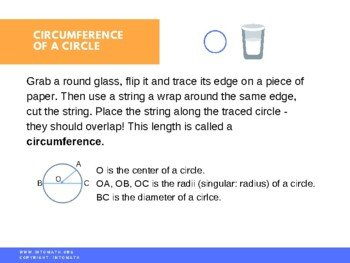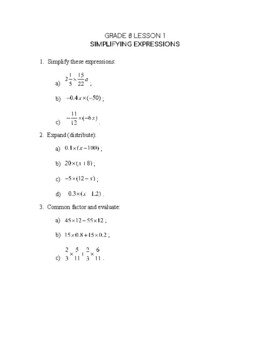CA\$2.00

In this lesson you will learn about scientific notation and its significance when working with very large and very small numbers.

Scientific notation is widely used in Physics, Astronomy and Chemistry.  For example, it could be used to express the volume of water in a large lake. Instead of writing all the zeros we multiply the number by a certain power of 10.

_________________________________

You will also learn how to calculate area and circumference of a circle.

You will discover the importance of the Pi number. Here are the first 100,000 digits of Pi.